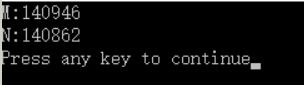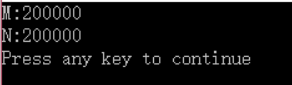Multithreading undoubtedly brings a lot of convenience and improves a lot of development efficiency, but it also brings a lot of problems.

Example:

``````DWORD WINAPI ThreadProc(LPVOID lPParameter);

int m = 0 ;
int n = 0 ;

int main( int argc, TCHAR* argv[], TCHAR* envp[])
{
int i = 0 ;
for (i = 0 ; i< 100000 ; i++ )
{
m ++ ;
n ++ ;
}
Sleep( 1 );
cout << " M: " <<m<< endl;
cout << " N: " <<n<< endl;
return  0 ;
}
{
int j = 0 ;
for (j = 0 ;j< 100000 ;j++ )
{
m ++ ;
n ++ ;
}
return  0 ;
}``````

What is the output of this program? According to common sense, it is not difficult to draw a conclusion: m and n are both 20000.

But what about the real results?The results are surprising, and the results are different every time ! Why does this happen?

When A thread accesses global resources, it cannot control B thread's access to global resources. When A takes out the memory data and puts it in the register operation, B also takes out the memory data and put it in the register operation, which may cause repeated operations! That is to say, when A is performing calculations, B can perform calculations before putting the calculation results back. So the final result is smaller than 200,000 and m and n are different.

In order to control the thread not to be disordered, it must be coordinated according to our intention, and it is inevitable to use thread control (mutual exclusion/synchronization) technology!

1. EVENT (event)

2. Critical Section (critical section)

3. Mutex (mutual exclusion)

4. Semaphore (semaphore)

EVENT (event)

Using events to synchronize threads is the most flexible . An event has two states: excited state and unexcited state. Also called the signaled state and the non-signaled state . There are two types of events: manual reset events and automatic reset events. After the manual reset event is set to the activated state, all waiting threads will be awakened and remain in the activated state until the program resets it to the inactivated state. After the auto reset event is set to the activated state, it will wake up "a" waiting thread, and then automatically return to the inactivated state. So it is ideal to synchronize two threads with automatic reset events . The corresponding class in MFC is CEvent. The constructor of CEvent creates an automatically reset event by default, and it is in an unfired state. Change the state of the event: SetEvent, ResetEvent.

Let's see how to use events to solve our actual problems:

``````DWORD WINAPI ThreadProc(LPVOID lPParameter);

int m = 0 ;
int n = 0 ;

int main( int argc, TCHAR* argv[], TCHAR* envp[])
{
HANDLE hEvent = NULL;
hEvent = CreateEvent(NULL,FALSE, FALSE,NULL);   // FALSE ×Ô¶¯ TRUE ÊÖ¶¯ FALSEÊÇ×Ô¶¯
WaitForSingleObject(hEvent,INFINITE);
int i = 0 ;
for (i=0 ;i< 100000 ;i++ )
{
m ++ ;
n ++ ;
}
Sleep( 1 );
cout << " M: " <<m<< endl;
cout << " N: " <<n<< endl ;
return  0 ;
}

{
HANDLE hEvent = NULL;
hEvent = (HANDLE)lPParameter;
int j = 0 ;
for (j= 0 ;j< 100000 ;j++ )
{
m ++ ;
n ++ ;
}
SetEvent(hEvent);
return  0 ;
}``````

Let's see if the result is correct:They are.

Critical Section

CRITICAL_SECTION is the fastest. Other kernel locks (events, mutexes) require thousands of CPU cycles each time they enter the kernel. The first advice for using critical regions is not to lock a resource for a long time.

The long time here is relative and depends on different procedures. For some control software, it can be several milliseconds, but for other programs, it can be several minutes. But after entering the critical zone, you must leave as soon as possible to release resources. If it is not released, what will happen? The answer is nothing. If the main thread (GUI thread) wants to enter a critical area that has not been released, the program will hang! One disadvantage of the critical section is: Critical Section is not a core object , and it is impossible to know whether the thread entering the critical section is alive or dead. If the thread entering the critical section hangs, the critical resource is not released , the system cannot know, and there is no way to release the critical section. Resources.

This shortcoming is made up for in the mutex (Mutex).

``````DWORD WINAPI ThreadProc(LPVOID lPParameter);

int m = 0 ;
int n = 0 ;

CRITICAL_SECTION cs;
int main( int argc, TCHAR* argv[], TCHAR* envp[])
{
InitializeCriticalSection( & cs);
int i = 0 ;
EnterCriticalSection( & cs);
for (i = 0 ;i< 100000 ;i++ )
{
m ++ ;
n ++ ;
}
LeaveCriticalSection( & cs);
Sleep( 1 );
cout << " M: " <<m<< endl;
cout << " N: " <<n<< endl;
return  0 ;
}
{
int j = 0 ;
EnterCriticalSection( &cs);
for (j = 0 ;j< 100000 ;j++ )
{
m ++ ;
n ++ ;
}
LeaveCriticalSection( & cs);
return  0 ;
}``````

The same way you can get the correct result!

Regarding the use of the critical section, it is a four-step problem: initialize the critical section --> enter the critical section --> leave the critical section --> destroy the critical section . Keep these four in mind and there will be no problems. One thing to note is: critical section and recursion should be used carefully, recursion in the critical section will cause deadlock!

Mutex (mutual exclusion)

The function of the mutex is very similar to the critical region. The difference is: Mutex spends more time than Critical Section, but Mutex is the core object (Event, Semaphore also), can be used across processes, and waiting for a locked Mutex can be set to TIMEOUT, unlike the Critical Section In that way, it is impossible to know the situation of the critical region, and it keeps waiting. Win32 functions are: create a mutex CreateMutex(), open a mutex OpenMutex(), release a mutex ReleaseMutex().

The ownership of Mutex does not belong to the thread that spawned it, but the last thread that waited for this Mutex (WaitForSingleObject, etc.) and has not yet performed the ReleaseMutex() operation. A thread owning a Mutex is like entering the Critical Section. Only one thread can own the Mutex at a time.

If a thread that owns a Mutex does not call ReleaseMutex() before returning, then the Mutex is discarded, but when other threads wait for this Mutex (WaitForSingleObject, etc.), they can still return and get a WAIT_ABANDONED_0 return value. It is unique to Mutex to be able to know that a Mutex is abandoned.

``````HANDLE hMutex = NULL;

int main( int argc, TCHAR* argv[], TCHAR* envp[])
{
hMutex = CreateMutex(NULL,TRUE,NULL);
int i = 0 ;
for (i = 0 ;i< 100000 ;i++ )
{
m ++ ;
n ++ ;
}
ReleaseMutex(hMutex);
Sleep( 1 );
cout<< " M: " <<m<< endl;
cout << " N: " <<n<< endl;
return  0 ;
}
{
WaitForSingleObject(hMutex,INFINITE);
int j = 0 ;
for (j= 0 ;j< 100000 ;j++ )
{
m ++ ;
n ++ ;
}
return  0 ;
}``````

Semaphore (semaphore)

Semaphore is the most historical synchronization mechanism. Semaphore is a key element to solve the producer/consumer problem. The Win32 function CreateSemaphore() is used to generate a semaphore. ReleaseSemaphore() is used to release the lock.

The current value of Semaphore represents the number of resources currently available. If the current value of Semaphore is 1, it means that there is another lock action that can succeed. If the current value is 5, it means there are five locking actions that can succeed. When calling Wait... and other functions to require locking, if the current value of Semaphore is not 0, Wait... returns immediately, and the number of resources is reduced by 1. When ReleaseSemaphore() is called, the number of resources is increased by 1, and it will not exceed the total number of resources initially set.

``````HANDLE hSemaphore = NULL;

int main( int argc, TCHAR* argv[], TCHAR* envp[])
{
hSemaphore = CreateSemaphore(NULL, 0 , 1 ,NULL);
int i = 0 ;
for (i = 0 ;i< 100000 ;i++ )
{
m ++ ;
n ++ ;
}
ReleaseSemaphore(hSemaphore, 1,NULL);
Sleep( 1 );
cout << " M: " <<m<< endl;
cout << " N: " <<n<< endl;
CloseHandle(hSemaphore);
return  0 ;
}

{
WaitForSingleObject(hSemaphore,INFINITE);
int j = 0 ;
for (j = 0 ;j< 100000 ;j++ )
{
m ++ ;
n ++ ;
}
return  0 ;
}``````